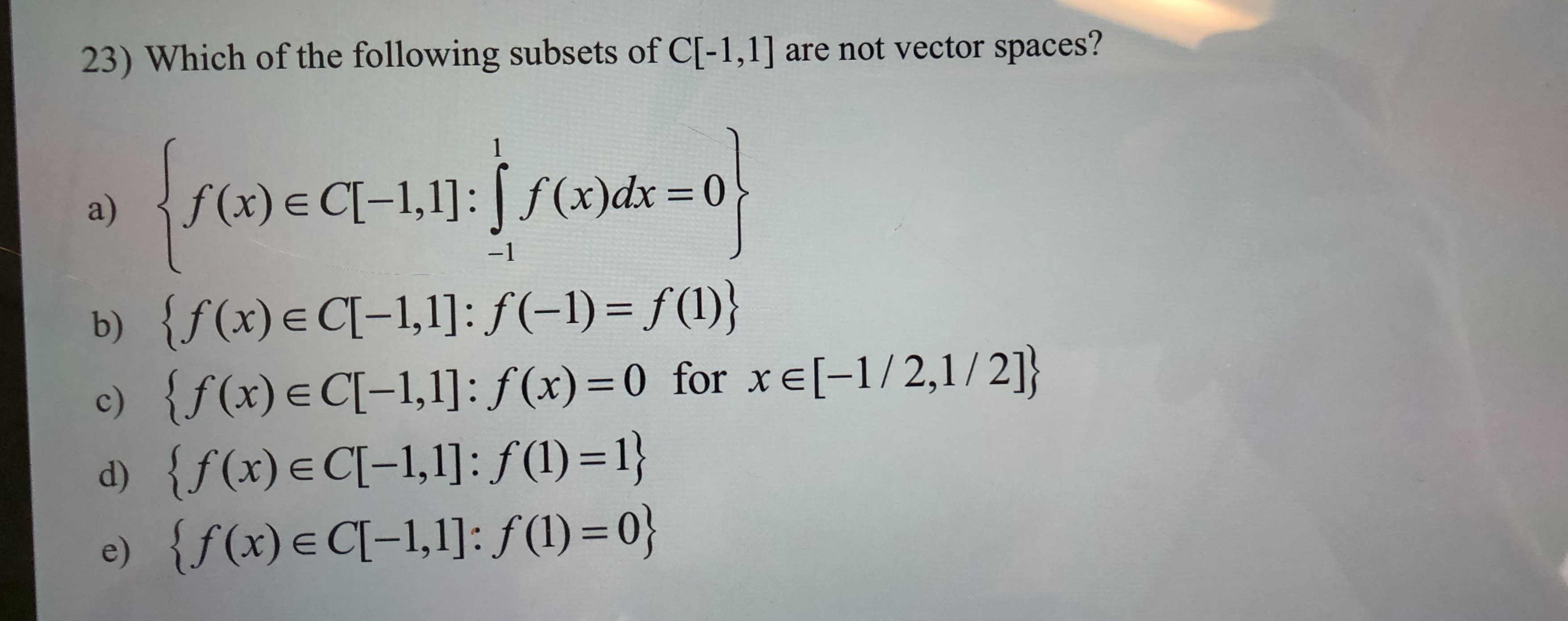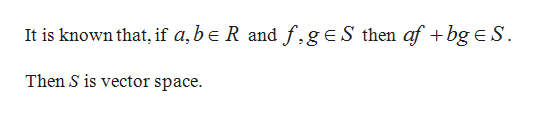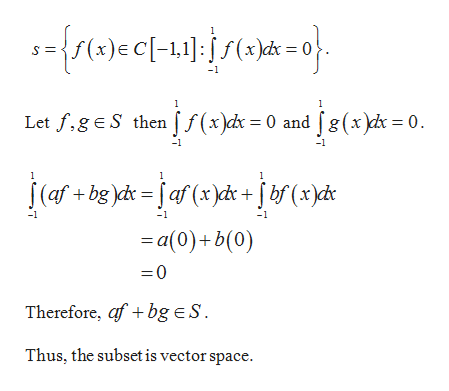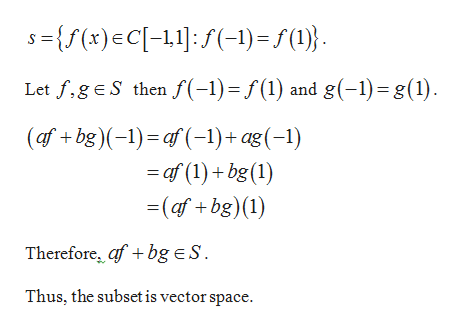# are not vector spaces?23) Which of the following subsets of C[-1,1]1(x)dx 0f(x) E CI-1,1]:a)-1{f(x)e C-1,1]: f(-1) = f(l)}{f (x) E C[-1,1]: f(x) 0 for xe[-1/2,1 / 2]}{f(x) e Cl-1,1]: f (1) = 1}e) {f(x)e CI-1,1]: f(l) = 0}b)c)d)

Questionhelp_outlineImage Transcriptioncloseare not vector spaces? 23) Which of the following subsets of C[-1,1] 1 (x)dx 0 f(x) E CI-1,1]: a) -1 {f(x)e C-1,1]: f(-1) = f(l)} {f (x) E C[-1,1]: f(x) 0 for xe[-1/2,1 / 2]} {f(x) e Cl-1,1]: f (1) = 1} e) {f(x)e CI-1,1]: f(l) = 0} b) c) d) fullscreen
check_circle

Step 1

Condition for vector space:help_outlineImage TranscriptioncloseIt is known that, if a,be R and f.geS then af + bg e S Then S is vector space. fullscreen
Step 2

a)

Consider the subset,help_outlineImage Transcriptionclose1 =f(x)e C[-1,1f(x)dh = 0 } 1 1 Let f,gE S then ff(x)d = 0 and fg(x)dc = 0 -1 1 (af+ bg d=faf (x)dx bf(xyd« -1 -1 a(0)b(0) =0 Therefore, af bg ES. Thus, the subset is vector space. fullscreen
Step 3

b)

Consider the s...help_outlineImage TranscriptioncloseS={f(x)EC[-1,1:f1)=S(1)} I Let f,geS then f(-1) = f(1) and g(-1) g(1) (afbg)-)af-1)+ ag(-1) -af (1)+bg(1) (af bg)() Therefore, afbgeS Thus, the subset is vector space. fullscreen

### Want to see the full answer?

See Solution

#### Want to see this answer and more?

Solutions are written by subject experts who are available 24/7. Questions are typically answered within 1 hour.*

See Solution
*Response times may vary by subject and question.
Tagged in

### Math